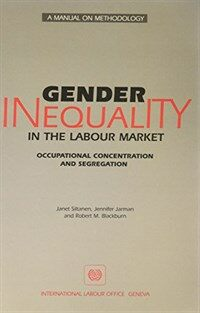> 상세정보

상세정보Gender inequality in the labour market : occupational concentration and segregation

자료유형
단행본
개인저자
Siltanen, Janet. Jarman, Jennifer. Blackburn, R. M. (Robert Martin).
단체저자명
ILO.
서명 / 저자사항
Gender inequality in the labour market : occupational concentration and segregation / Janet Siltanen, Jennifer Jarman, and Robert M. Blackburn.
발행사항
Geneva :   International Labour Office,   c1995.
형태사항
xi, 124 p. : ill. ; 24 cm.
ISBN
9221091368 :
일반주기
At head of title: A manual on methodology.
서지주기
Includes bibliographical references (p. 117-119).
일반주제명
Sex discrimination in employment --Data processing --Methology.
 000 01134camuuu200325 a 4500 001 000000576263 003 OCoLC 005 19971226101304.0 008 950829s1995 sz a b i000 0 eng d 010 ▼a 96117513 020 ▼a 9221091368 : ▼c 22.5 SF 040 ▼a MoSU-L ▼c DLC ▼d SLU ▼d UIU ▼d 211009 042 ▼a lccopycat 049 1 ▼l 481033711 ▼f UN-IL 050 0 0 ▼a HD6060 ▼b .S55 1995 082 0 0 ▼a 331.4133 ▼2 20 090 ▼a 331.4133 ▼b gi 110 2 ▼a ILO. 245 1 0 ▼a Gender inequality in the labour market : ▼b occupational concentration and segregation / ▼c Janet Siltanen, Jennifer Jarman, and Robert M. Blackburn. 260 ▼a Geneva : ▼b International Labour Office, ▼c c1995. 300 ▼a xi, 124 p. : ▼b ill. ; ▼c 24 cm. 500 ▼a At head of title: A manual on methodology. 504 ▼a Includes bibliographical references (p. 117-119). 650 0 ▼a Sex discrimination in employment ▼x Data processing ▼x Methology. 700 1 ▼a Siltanen, Janet. 700 1 ▼a Jarman, Jennifer. 700 1 ▼a Blackburn, R. M. ▼q (Robert Martin). 710 2 ▼a International Labour Office. 740 0 1 ▼a Manual on methodology.

소장정보

No. 소장처 청구기호 등록번호 도서상태 반납예정일 예약 서비스
No. 1 소장처 청구기호 331.4133 gi 등록번호 481033711 도서상태 대출불가(자료실) 반납예정일 예약 서비스

컨텐츠정보

목차

CONTENTS
Preface = ⅴ
Authors' acknowledgements = ⅶ
1. Introduction = 1
Aims and purposes of the manual = 1
Organization of the manual = 3
Clarifying concepts and definitions = 3
Segregation = 4
Concentration = 5
Problems of measurement = 6
The quality of data = 7
Consistency in data collection and recording = 7
Definitions of the labour force = 7
Definitions that distinguish forms of employment = 8
Consistency in occupational classification schemes = 8
Coding data to more than one occupational classification scheme = 9
Backward and forward aggregating = 9
Using a constant subset of occupations = 10
Level of data aggregation = 11
Industrial classification = 12
Data quality: Final remarks = 12
A vertical dimension = 13
Identifying a suitable measurement procedure = 14
2. Simple descriptive procedures = 17
Introduction = 17
The sex composition of an occupation = 18
The example data = 18
Step 1. Sex composition of occupations = 18
Step 2. Distribution of occupations according to percentage female = 23
Step 3. Analysis of the extremes = 24
Step 4. Analysis of the middle of the distribution = 26
Step 5. Further analysis of the middle of the distribution = 27
Step 6. Male and female dominance in occupations compared to the sex composition of the employed labour force = 27
Step 9. Concentration patterns across time periods = 32
A note of caution = 33
Conclusion = 34
3. Marginal Matching: A procedure for analysing trends in occupational segregation = 35
Introduction = 35
Occupational segregation and Marginal Matching : General information = 36
Extending the use of the Marginal Matching procedure = 39
Summary = 40
Instructions for finding the level of occupational segregation = 41
Program sets for SPSS = 41
A program set for Lotus 1-2-3 = 42
Worksheets and definitions of symbols = 42
Analysing trends over time = 43
SPSS program set 1: Instructions for data where the occupation is the unit of analysis = 44
Lotus 1-2-3 program set: Instructions for data where the occupation is the unit of analysis = 60
SPSS program set 2: Instructions for data where the individual is the unit of analysis = 72
4. Evaluating measures of gender segregation = 87
Gender segregation measures = 87
The mathematical forms of the segregation indices = 89
The Index of Dissimilarity (ID) = 90
The Sex Ratio Index (SR) = 91
The WE Index (WE) = 91
The Gini Coefficient (G) = 92
Segregation measures as statistics of association = 93
Marginal Matching (MM) - Mathematical form and relation to Basic Segregation Table = 95
Deciding on a measure of occupational gender segregation = 96
The conceptualization of occupational segregation, and its relation to the scale of measured values = 97
The insensitivity of measured segregation values to factors other than segregation = 101
The advantage of Marginal Matching = 106
Conclusion = 107
Instructions for finding other indices of segregation = 107
SPSS program set 1: Instructions for data where the occupation is the unit of analysis = 108
Lotus 1-2-3 program set: Instructions for data where the occupation is the unit of analysis = 111
SPSS program set 2: Instructions for data where the individual is the unit of analysis = 113
References = 117

관련분야 신착자료

팽혜영 (2021)

박병규 (2021)

한국직업능력개발원 (2021)

김영선 (2022)

어둠의 시대 불꽃이 되어 : 1970년대 민주노동운동의 현장 : 그 삶과 투쟁의 기록

70년대민주노동운동동지회 (2021)

방준호 (2021)

휴먼 엣지 : IQ,재능,운,환경을 뛰어넘는 강력한 4C의 힘

Orme, Greg (2021)

이다혜 (2021)

Career and family : women's century-long journey toward equity

Goldin, Claudia Dale (2021)

강승욱 (2021)

전혜원 (2021)

전국금속노동조합 (2021)

이소진 (2021)

양승광 (2021)

박종천 (2021)

남윤성 (2021)

커리어 그리고 가정 : 평등을 향한 여성들의 기나긴 여정

Goldin, Claudia Dale (2021)

아이폰을 위해 죽다 : 애플, 폭스콘, 그리고 중국 노동자의 삶

Chan, Jenny (2021)

노동경제학

Borjas, George J. (2021)